Gravitational force (internal energy-exchange imbalance)
The figure at left shows a box within which photons b and t are emitted from the bottom B and top T and travel to the opposite ends where they are absorbed. In the absence of massive body m, the energy of photon b transferred from B to T is the same as the energy of photon t transferred from T to B. However, according to Premise II of the qm view, a massive body causes a change in the qm which slows the rate of energy transfer through the qm. Specifically, a massive body m slows the energy-exchange rate in any physical system (e.g. atom) in its vicinity according to the following equation, where m is the mass of the massive body in kga,is the distance from the massive body in LS, and G is the Newtonian gravitational constant. The gravity-causing physical change ratio (rg) is the ratio of the rate of round-trip energy exchange in the body to the rate in the absense of m, much like rv is the ratio of the rate of round-trip energy exchange in a body moving through the qm to the at-rest rate.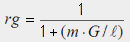Just as the rates of physical processes are affected by rv, they are similarly affected by rg. For example, a clock located at sea level runs slower than one located 1000 m higher, and we can use the rg equation to determine the difference in clock rates (about 1E−13). Similarly, the frequencies of photons b and t depend on rg at B (rgB) and rg at T (rgT). And the difference between the emission energies of b and t (Δeg) is a function of Planck's constant h, the emission frequency f when rg=1, and the difference between rgT and rgB, as follows.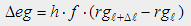We will use the rg and Δeg equations above to calculate Δeg for a box 3 ma long (i.e. Δ=1E−8 LS) at Earth's surface. Earth's mass is 5.98E24 kg, Earth's radius is about 6.38E6 ma or about .02126666 LS, and when distance is in LS units, G=2.47E−36. Therefore, rgB=1/[1+(5.98E24·2.47E−36/.02126666)] = (1−6.9454253699E−10) and rgT=1/[1+(5.98E24·2.47E−36/.02126667) = (1−6.9454221040E−10). The difference between rgT and rgB is only 3.2659E−16, and Δeg=h·f·3.2659E−16 J (a quantity we will be using on the next page). As shown in the figure at left, this small energy-exchange imbalance between B and T in the box, Δeg, is due to lower energy photons emitted at B (red dots) transferring less energy to T than the higher energy photons emitted at T (blue dots) transfer to B. On the next page we determine the acceleration of a box necessary to create the same energy-exchange imbalance.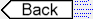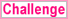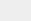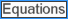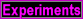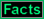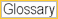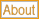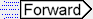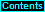To page: 1 2 3 3a 4 5 6 7 8 9 10 11 12 13 14 15 16 17 18 19 20 21 22 23 24 25 26 27 28 29 29a 30 31 32